# Knowledge Base

## Solution 30931: Computing the Doolittle LU (lower-upper) Decomposition of a Matrix on the TI-Nspire™ Family and TI-Nspire Computer Software.

### How do I compute the LU decomposition of a matrix on the TI-Nspire family or TI-Nspire Computer software?

The instructions below demonstrate how to compute the Doolittle (lower-upper) decomposition of a real or complex matrix on the TI-Nspire family and TI-Nspire Computer Software.

Matrix for this example is below: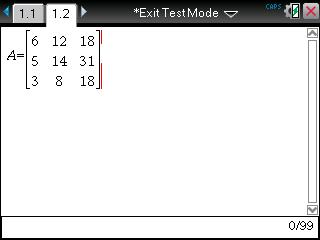To enter the matrix A into the Matrix &Vector:

• Press [menu], press 7: Matrix & Vector.
• Press 1: Create and press 1: Matrix.
• Enter 3 for the Number of rows.
• Enter 3 for the Number of columns.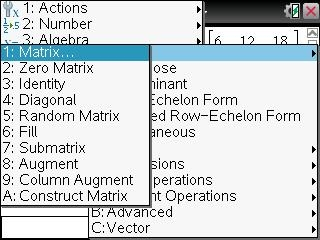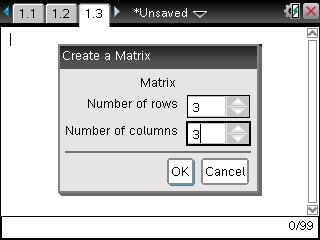• Press , [tab], , [tab], , [tab]
• Press , [tab], , [tab], , [tab]
• Press , [tab], , [tab], , [tab]
• Press [ctrl], [sto]
• Press [A], [enter]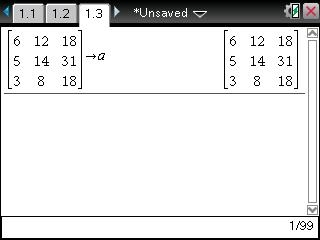To calculate the LU (lower-upper) decomposition of the matrix:

• Press 7: Matrix & Vector
• Press 2: LU Decomposition

Note: You can also access the LU by pressing catalog and down to LU for the full syntax.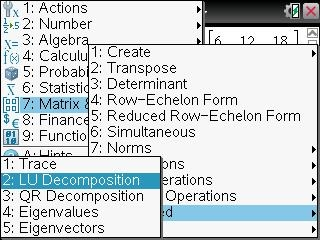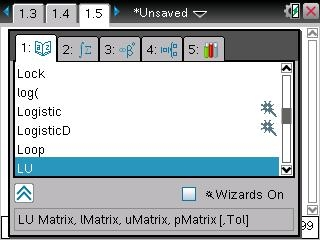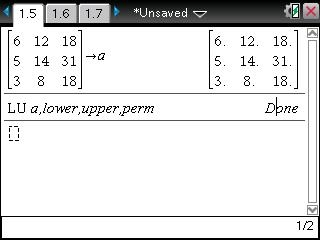The command to calculate the Doolittle LU (lower-upper) decomposition of a real or complex matrix must be done before calculating . Please follow the steps below:

• Type: LU a, lower, upper, perm
• Press [enter] it will say done

The lower triangular matrix is stored in lMatrix, the upper triangular matrix in uMatrix, and the permutation matrix (which describes the row swaps done during the calculation) in pMatrix.

To calculate lower matrix:
• Type lower and press [enter] to get the answer.

To calculate upper matrix:
• Type upper and press [enter] to get the answer.

To calculate permutation matrix:
• Type perm and press [enter] to get the answer.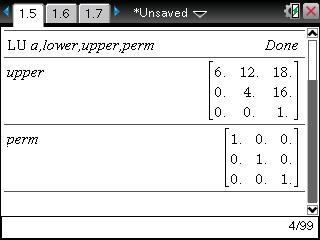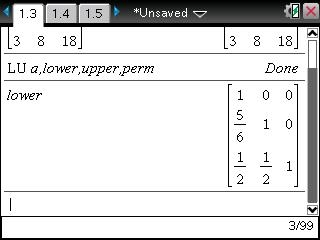lMatrix*uMatrix=pMatrix*matrix
Optionally, any matrix element is treated as zero if its absolute value is less than Tolerance. This tolerance is used only if the matrix has floating point entries and does not contain any symbolic variables that have not been assigned a value. Otherwise, Tol is ignored.